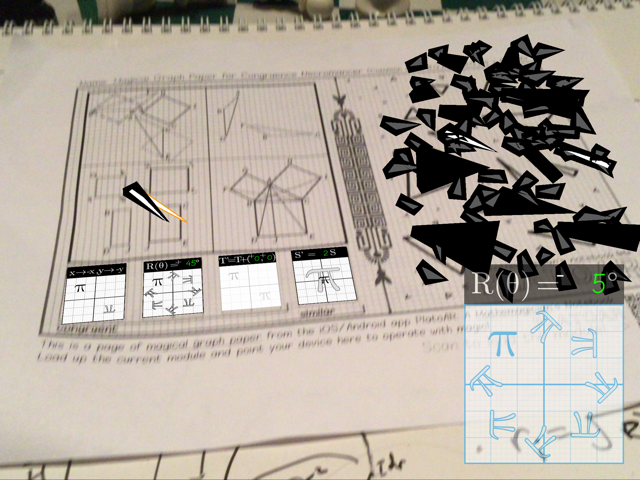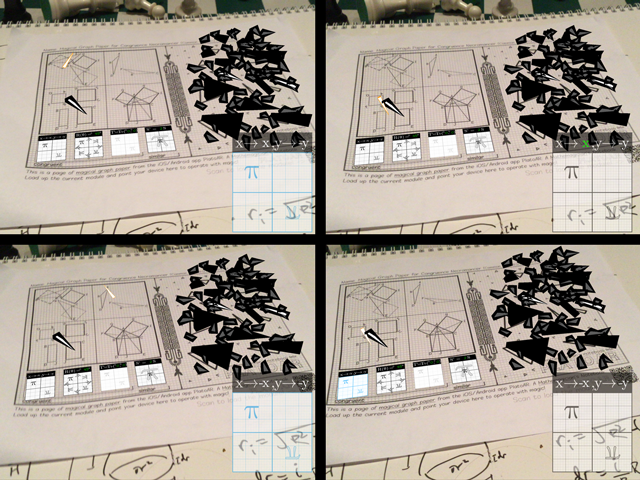## Congruence Necromancer

This module addresses Common Core 8.G.A.2 + 8.G.A.4 + 8.G.B.6.

The main interactivity element involves transforming one congruent triangle to match the other, with the goal of "necromancing" a shattered insect made of triangles — "back to life".

Students apply transformations by utilizing operator methodology. Press each corresponding button to either mirror, rotate, translate, or scale the currently glowing triangle. Use the right combination of transforms for it to perfectly match the black triangle. The video tutorial example shows how each sample triangle is transformed using each operator.Screen overlay interface is shown once the rotation button is pressed on paper.

When an operator on paper is pressed, a screen overlay version of this operator appears. Both the screen-overlay and on-paper versions allow for changing the operation parameters - such as rotation degrees, mirror negation factors, translation coordinates, and dilation scale. Here are the allowed operation parameters:

• Mirror axes: toggling the "-" sign before x will flip the x coordinate, or reflect across the y axis, similarly toggling the "-" sign before the y will flip the y coordinate, or reflect across the x axis. Toggling both will reflect diagonally, from Q1 to Q3 for example. The identity operation occurs when both signs are positive "+".
• Rotation degree: any positive number (actual input is rounded to the nearest 5 degree). There is a toggle for a "+" or "-" sign preceding the number.
• Translation coordinates: any integer (non-integers will be rounded) less than 10
• Dilation factor: any positive integer (non-integers will be rounded)Examples of mirror operations via changing corresponding negation signs

[Under Development] Pythagorean triangles transformations sub-module.